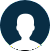#### You have questions? We got answers!The coordinates of the midpoints of the sides of a triangle are l(0,1),m(4,0),& n(2,-2).find the coordinates of the vertices of the triangle?

Science & Mathematics by Anonymous 2018-08-31 03:32:14

Social Science

The coordinates of the midpoints of the sides of a triangle are l(0,1),m(4,0),& n(2,-2).find the coordinates of the vertices of the triangle?

No response

ReCapcha
Not a bot